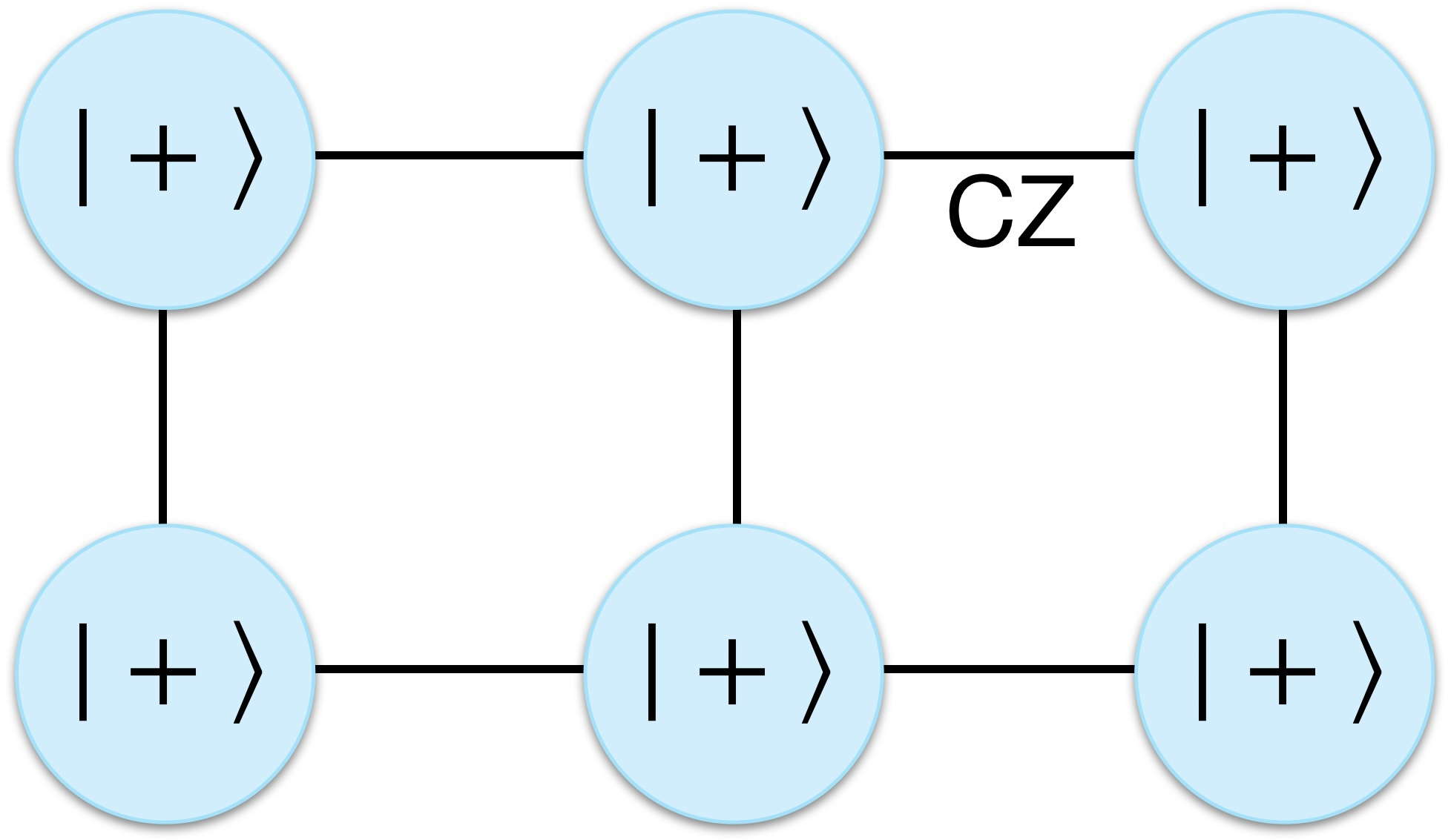# An introduction to graph states

#### What is a graph state?

One especially useful class of quantum states is graph states, also known as cluster states. As the name suggests, a graph state |G\rangle is associated with a graph,

G=(V,E),

where the vertices (v\in V) represent qubits initialised into the

|+\rangle = (|0\rangle+|1\rangle)/\sqrt{2}

state, and edges (e\in E) represent the application of controlled-phase (CZ) gates between respective vertices, where,

\mathrm{CZ}=\mathrm{diag}(1,1,1,-1).

A graph state can therefore be expressed as,

|G\rangle = \left[ \prod_{e\in E} \mathrm{CZ}_e \right] |+\rangle^{\otimes |V|}.

Since CZ gates are diagonal and therefore commute with one another, the order in which they are applied is irrelevant, meaning there is great flexibility in the preparation of graph states and room for parallelisation in the application of the required CZ gates.A graph state is defined with respect to a graph, where vertices represent qubits initialised into the |+\rangle state and edges represent the application of CZ gates between qubits. Since CZ gates commute, the order in which they are applied is irrelevant.
Continue reading An introduction to graph states

# How do photonic Bell measurements work?

Entangling Bell measurements are an essential ingredient in many photonic quantum technologies. In optical quantum computing they are employed as fusion gates to create edges in graph states, while in quantum communications protocols they may be used to implement entanglement swapping in quantum repeater networks (entanglement distribution networks) for extending the range of entanglement links.

In this post I’ll describe how this very simple optical circuit works and address some of the nuances and common misconceptions surrounding it.

Continue reading How do photonic Bell measurements work?

# A general framework for the composition of quantum homomorphic encryption & quantum error correction

A new paper on the arXiv with Yingkai Ouyang on composing quantum homomorphic encryption with quantum error correction, necessary for large-scale, secure, cloud quantum computing.

Abstract: Two essential primitives for universal, cloud-based quantum computation with security based on the laws of quantum mechanics, are quantum homomorphic encryption with information-theoretic security and quantum error correction. The former enables information-theoretic security of outsourced quantum computation, while the latter allows reliable and scalable quantum computations in the presence of errors. Previously these ingredients have been considered in isolation from one another. By establishing group-theoretic requirements that these two ingredients must satisfy, we provide a general framework for composing them. Namely, a quantum homomorphic encryption scheme enhanced with quantum error correction can directly inherit its properties from its constituent quantum homomorphic encryption and quantum error correction schemes. We apply our framework to both discrete- and continuous-variable models for quantum computation, such as Pauli-key and permutation-key encryptions in the qubit model, and displacement-key encryptions in a continuous-variable model based on Gottesman-Kitaev-Preskill codes.